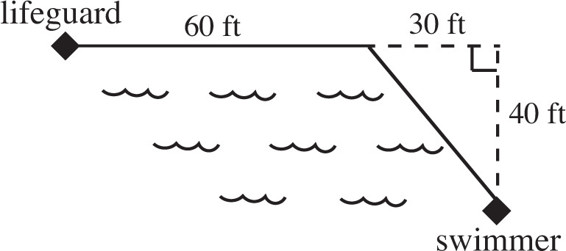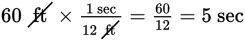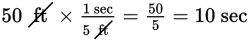# SAT Math Multiple Choice Question 507: Answer and Explanation

### Test Information

Question: 507

12.As shown in the figure above, a lifeguard sees a struggling swimmer who is 40 feet from the beach. The lifeguard runs 60 feet along the edge of the water at a speed of 12 feet per second. He pauses for 1 second to locate the swimmer again, and then dives into the water and swims along a diagonal path to the swimmer at a speed of 5 feet per second. How many seconds go by between the time the lifeguard sees the struggling swimmer and the time he reaches the swimmer?

• A. 16
• B. 22
• C. 50
• D. 56

Explanation:

A

Difficulty: Hard

Category: Additional Topics in Math / Geometry

Strategic Advice: In this question, information is given in both the diagram and the text. You need to relate the text to the diagram, one piece of information at a time, to calculate how long the lifeguard ran along the beach and how long he swam. Before you find the swim time, you need to know how far he swam.

Getting to the Answer: Whenever you see a right triangle symbol in a diagram, you should think Pythagorean theorem or, in this question, special right triangles. All multiples of 3-4-5 triangles are right triangles, so the length of the lifeguard's swim is the hypotenuse of a 30-40-50 triangle, or 50 feet. Add this number to the diagram. Now calculate the times using the distances and the speeds given. Don't forget the 1 second that the lifeguard paused.

Run time =Pause time = 1 sec

Swim time =Total time = 5 + 1 + 10 = 16 seconds